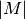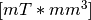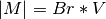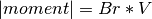# magpylib.source.moment package¶

This subpackge includes magnetic moment classes for field computation. Currently it includes only the Dipole (magnetisches dipol moment) class.

class `magpylib.source.moment.``Dipole`(moment=(0.0, 0.0, 0.0), pos=(0.0, 0.0, 0.0), angle=0.0, axis=(0.0, 0.0, 1.0))[source]

Bases: `magpylib._lib.classes.base.MagMoment`

This class represents a magnetic dipole. The dipole is constructed such that its momentis given inand corresponds to the moment of a cuboid magnet with remanence field Br and Volume V such that. Scalar input is either integer or float. Vector input format can be either list, tuple or array of any data type (float, int).

Parameters: moment (vec3 [mT]) – Set magnetic dipole moment in units of [mT*mm^3]. pos=[0,0,0] (vec3 [mm]) – Set position of the moment in units of [mm]. angle=0.0 (scalar [deg]) – Set angle of orientation of the moment in units of [deg]. axis=[0,0,1] (vec3 []) – Set axis of orientation of the moment.
`moment`

Magnetic dipole moment in units of [mT*mm^3] (of a cuboid magnet.)

Type: arr3 [mT]
`position`

Position of the moment in units of [mm].

Type: arr3 [mm]
`angle`

Angle of orientation of the moment in units of [deg].

Type: float [deg]
`axis`

Axis of orientation of the moment.

Type: arr3 []

Examples

```>>> magpylib as magpy
>>> mom = magpy.source.moment.Dipole(moment=[0,0,1000])
>>> B = mom.getB([1,0,1])
>>> print(B)
[0.33761862  0.  0.11253954]
```

Note

The following Methods are available to all source objects.

`getB`(pos)[source]

This method returns the magnetic field vector generated by the source at the argument position pos in units of [mT]

Parameters: pos (vec3 [mm] Position or list of Positions where magnetic field) – should be determined. magnetic field vector – position pos generated by the source in units of [mT]. arr3 [mT] Magnetic field at the argument
`move`(displacement)

This method moves the source by the argument vector displacement. Vector input format can be either list, tuple or array of any data type (float, int).

Parameters: displacement (vec3 [mm]) – Set displacement vector None

Example

```>>> from magpylib import source
>>> pm = source.magnet.Sphere(mag=[0,0,1000],dim=1,pos=[1,2,3])
>>> print(pm.position)
[1. 2. 3.]
>>> pm.move([3,2,1])
>>> print(pm.position)
[4. 4. 4.]
```
`rotate`(angle, axis, anchor='self.position')

This method rotates the source about axis by angle. The axis passes through the center of rotation anchor. Scalar input is either integer or float. Vector input format can be either list, tuple or array of any data type (float, int).

Parameters: angle (scalar [deg]) – Set angle of rotation in units of [deg] axis (vec3 []) – Set axis of rotation anchor (vec3 [mm]) – Specify the Center of rotation which defines the position of the axis of rotation. If not specified the source will rotate about its own center. None

Example

```>>> from magpylib import source
>>> pm = source.magnet.Sphere(mag=[0,0,1000], dim=1)
>>> print(pm.position, pm.angle, pm.axis)
[0. 0. 0.] 0.0 [0. 0. 1.]
>>> pm.rotate(90, [0,1,0], anchor=[1,0,0])
>>> print(pm.position, pm.angle, pm.axis)
[1., 0., 1.] 90.0 [0., 1., 0.]
```
`setOrientation`(angle, axis)

This method sets a new source orientation given by angle and axis. Scalar input is either integer or float. Vector input format can be either list, tuple or array of any data type (float, int).

Parameters: angle (scalar [deg]) – Set new angle of source orientation. axis (vec3 []) – Set new axis of source orientation. None

Example

```>>> from magpylib import source
>>> pm = source.magnet.Sphere(mag=[0,0,1000],dim=1)
>>> print([pm.angle,pm.axis])
[0.0, array([0., 0., 1.])]
>>> pm.setOrientation(45,[0,1,0])
>>> print([pm.angle,pm.axis])
[45.0, array([0., 1., 0.])]
```
`setPosition`(newPos)

This method moves the source to the position given by the argument vector newPos. Vector input format can be either list, tuple or array of any data type (float, int)

Parameters: newPos (vec3 [mm]) – Set new position of the source. None

Example

```>>> from magpylib import source
>>> pm = source.magnet.Sphere(mag=[0,0,1000],dim=1)
>>> print(pm.position)
[0. 0. 0.]
>>> pm.setPosition([5,5,5])
>>> print(pm.position)
[5. 5. 5.]
```